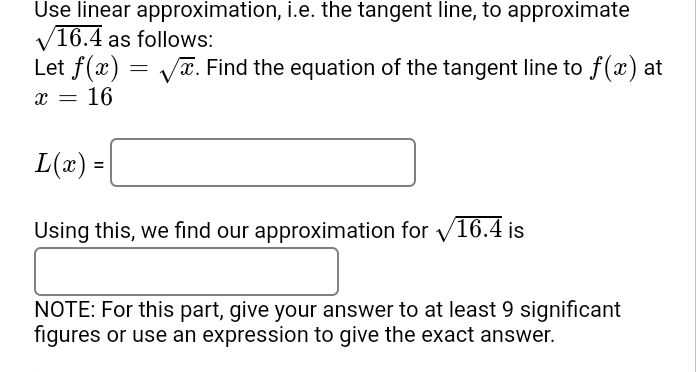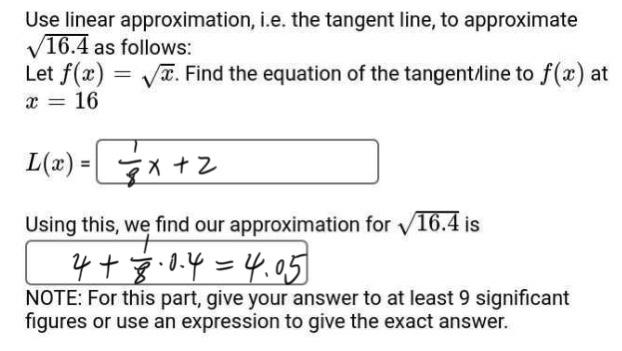### Still have math questions?

Algebra
QuestionUse linear approximation, i.e. the tangent line, to approximate $$\sqrt { 16.4 }$$ as follows:

Let $$f ( x ) = \sqrt { x }$$ . Find the equation of the tangent line to $$f ( x )$$ at $$x = 16$$

$$L ( x ) = \square$$

Using this, we find our approximation for $$\sqrt { 16.4 }$$ is

NOTE: For this part, give your answer to at least $$9$$ significant figures or use an expression to give the exact answer.